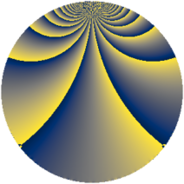Properties

 Label 1440.1.cdLevel $1440$ Weight $1$ Character orbit 1440.cd Rep. character $\chi_{1440}(929,\cdot)$ Character field $\Q(\zeta_{6})$ Dimension $8$ Newform subspaces $1$ Sturm bound $288$ Trace bound $0$

Related objects

Defining parameters

 Level: $$N$$ $$=$$ $$1440 = 2^{5} \cdot 3^{2} \cdot 5$$ Weight: $$k$$ $$=$$ $$1$$ Character orbit: $$[\chi]$$ $$=$$ 1440.cd (of order $$6$$ and degree $$2$$) Character conductor: $$\operatorname{cond}(\chi)$$ $$=$$ $$45$$ Character field: $$\Q(\zeta_{6})$$ Newform subspaces: $$1$$ Sturm bound: $$288$$ Trace bound: $$0$$

Dimensions

The following table gives the dimensions of various subspaces of $$M_{1}(1440, [\chi])$$.

Total New Old
Modular forms 40 8 32
Cusp forms 8 8 0
Eisenstein series 32 0 32

The following table gives the dimensions of subspaces with specified projective image type.

$$D_n$$ $$A_4$$ $$S_4$$ $$A_5$$
Dimension 8 0 0 0

Trace form

 $$8 q + O(q^{10})$$ $$8 q - 4 q^{21} + 4 q^{25} - 12 q^{29} - 8 q^{45} + 4 q^{49} + 4 q^{69} + 4 q^{81} + O(q^{100})$$

Decomposition of $$S_{1}^{\mathrm{new}}(1440, [\chi])$$ into newform subspaces

Label Dim. $$A$$ Field Image CM RM Traces $q$-expansion
$a_{2}$ $a_{3}$ $a_{5}$ $a_{7}$
1440.1.cd.a $8$ $0.719$ $$\Q(\zeta_{24})$$ $D_{12}$ $$\Q(\sqrt{-5})$$ None $$0$$ $$0$$ $$0$$ $$0$$ $$q-\zeta_{24}^{7}q^{3}-\zeta_{24}^{10}q^{5}+(-\zeta_{24}-\zeta_{24}^{3}+\cdots)q^{7}+\cdots$$